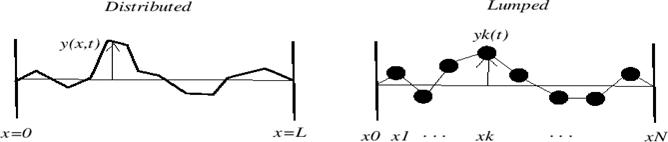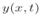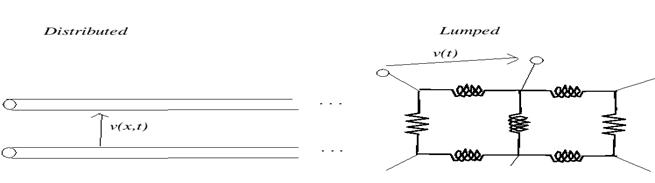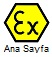A lumped system is one in which the dependent variables of interest are a function of time alone. In general, this will mean solving a set of ordinary differential equations (ODEs)

Lumped circuit is a circuit where impedance is not the function of length or distance from the source. Generally, at low frequency like 50Hz or pure DC, it is possible to see the impedance separately. But when the frequency increases, like in transmission lines, it is not possible to identify where is resistance, inductance or capacitance. They vary with the distance. The conductor itself shows impedance as a function of its length. At this moment, the laws for lumped circuit like Kirchoff's law is not applicable but Ohms law is applicable (J=Sigma*E)

A distributed system is one in which all dependent variables are functions of time and one or more spatial variables. In this case, we will be solving partial differential equations (PDEs) Lumped systems are those systems in which electrical properties like R, L, C etc are assumed to be located on a small space of the circuit. these systems are applicable for low frequency application. On the other hand distributed systems assume that electrical properties R, L, C etc are distributed across the entire circuit. these systems are applicable to for high (microwave) frequency application. Unlike the lumped element model, it assumes non-uniform current along each branch and non-uniform voltage along each node.

For example, consider the following two systems:The first system is a distributed system, consisting of an infinitely thin string, supported at both ends; the dependent variable, the vertical position of the string is indexed continuously in both space and time.

The second system, a series of beads'' connected by massless string segments, constrained to move vertically, can be thought of as a lumped system, perhaps an approximation to the continuous string.

For electrical systems, consider the difference between a lumped RLC network and a transmission line